## Creating Maps with GeoPandas¶

GeoPandas comes with built-in functions for visualizing geospatial data and creating maps. It uses the very powerful matplotlib library to do the plotting. If you are not familiar with matplotlib, check out this introductory tutorial.

This notebook shows how we can create visualizaion using the datasets from the Working with GeoPandas exercise.

In :
import geopandas as gpd
import os
data_pkg_path = 'data'
filename = 'karnataka.gpkg'
path = os.path.join(data_pkg_path, filename)


### Matplotlib Basics¶

Before we start using matplotlib inside a Jupyter notebook, it is useful to set the matplotlib backend to inline. This setting makes the matplotlib graphs included in your notebook, next to the code. We use the magic function %matplotlib to achieve this.

In :
%matplotlib inline
import matplotlib.pyplot as plt


It is important to understand the 2 matplotlib objects

• Figure: This is the main container of the plot. A figure can contain multiple plots inside it
• Axes: Axes refers to an individual plot or graph. A figure contains 1 or more axes.

We can now work on creating a figure with multiple axes - each with a different rendering on a map layer.

### Rendering Map Layouts¶

The subplots() function creates one or more plots within the figure. You can design a map layout with multiple rows/columns. In the code below, we create a map with 1 row and 3 columns. Using the set_size_inches() function, we set the size of the map to 15in x 7in.

In :
fig, axes = plt.subplots(1, 3)
fig.set_size_inches(15,7)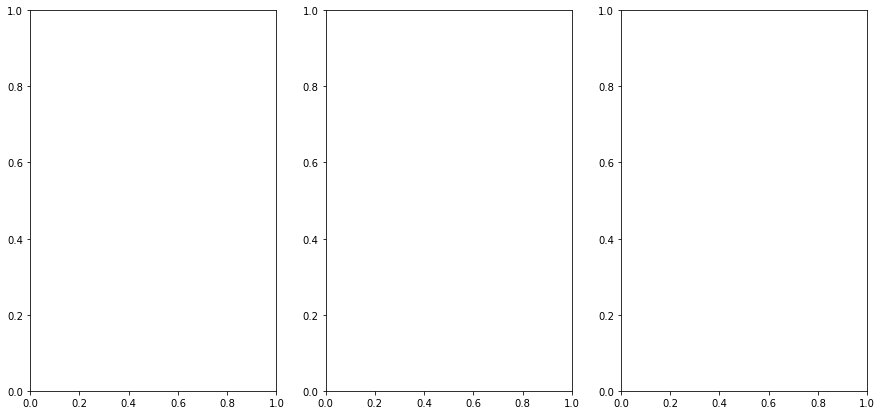The subplots() function returns 2 items. The figure and a tuple with all the axes within the figure. As we have 3 axes, we unpack them into separate variables

In :
ax0, ax1, ax2 = axes


GeoDataFrame objects have a plot() method that uses pyplot and creates a plot. We supply the ax object to the function so the resulting plot is displayed in the Axes created previously. Here we add the districts polygon layer into the ax0 object - which refers to the first subplot.

In :
districts.plot(ax=ax0, linewidth=1, facecolor='none', edgecolor='#252525')
fig

Out: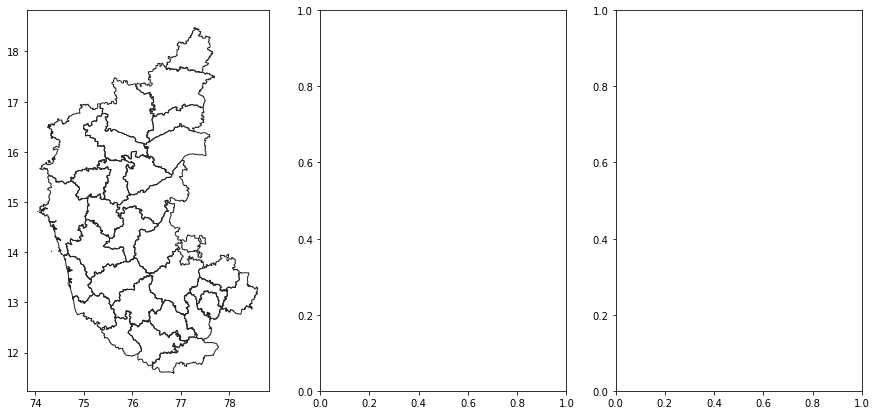<Figure size 432x288 with 0 Axes>

Similarly, we add the roads layer to the second axes.

In :
roads.plot(ax=ax1, linewidth=0.4, color='#2b8cbe')
fig

Out: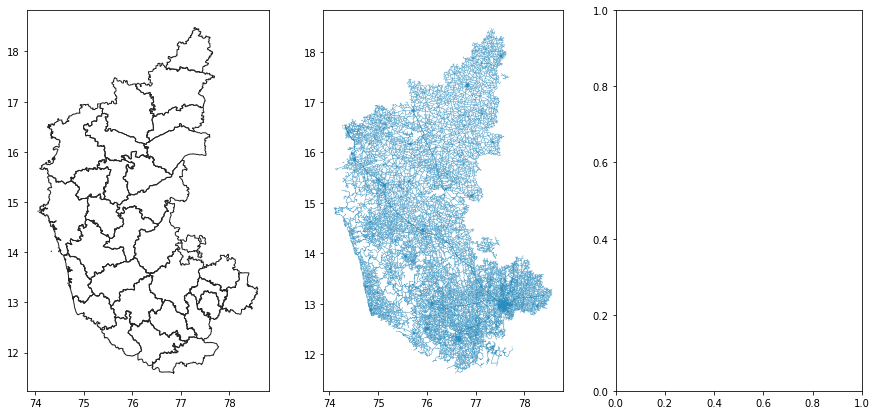<Figure size 432x288 with 0 Axes>

Lastly, we add the national_highways layer to the third axes.

In :
national_highways.plot(ax=ax2, linewidth=1, color='#de2d26')
fig

Out: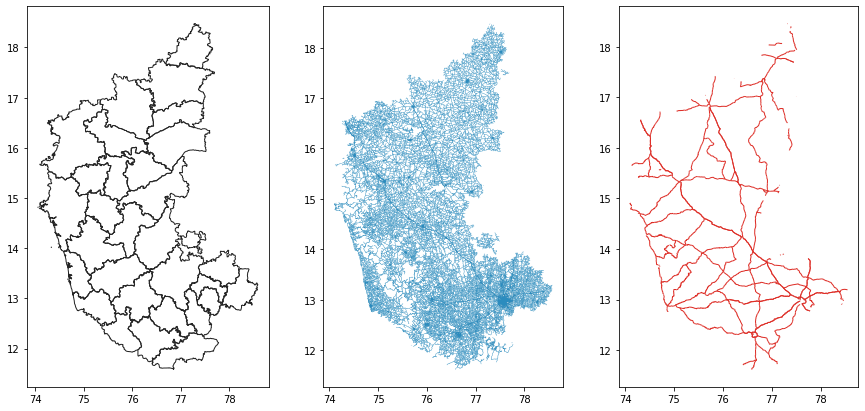<Figure size 432x288 with 0 Axes>

We can turn off the coordinates display on the X-axis and Y-axis using plt.axis('off'). It is useful to set a title to each map. The set_title() function adds the title to the approproate axes. We specify a negative y parameter to place the title at the bottom of the map instead of top.

In :
ax0.axis('off')
ax0.set_title('Karnataka Districts', y=-0.1)
ax1.axis('off')
ax2.axis('off')
ax2.set_title('Karnataka National Highways', y=-0.1)
fig

Out: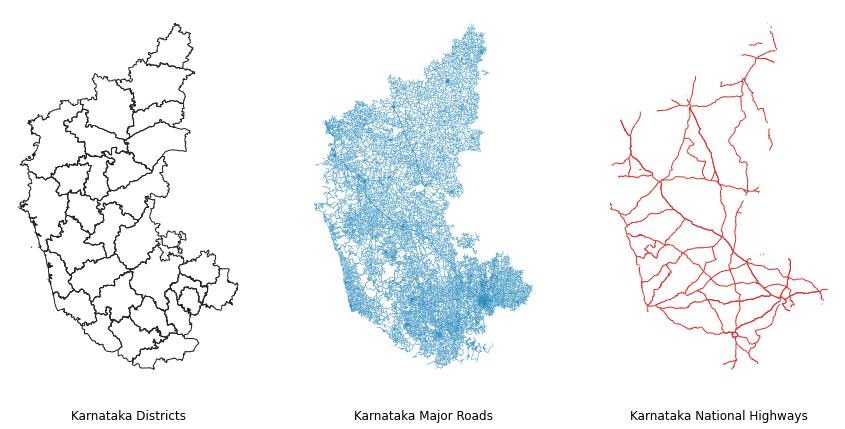Now that our map is ready, we can save the map to the computer using the savefig() function.

In :
output_filename = 'map_layout.png'
output_dir = 'output'
output_path = os.path.join(output_dir, output_filename)

if not os.path.exists(output_dir):
os.mkdir(output_dir)

fig.savefig(output_path, dpi=300)


### Creating A Map with Multiple Layers¶

If we want to display multiple layers, we simply create new plots on the same Axes. Here we create a figure with a single axes and add the districts,roads and national_highways layers to the same axes.

In :
fig, ax = plt.subplots()
fig.set_size_inches(10,15)

plt.axis('off')

districts.plot(ax=ax, linewidth=1, facecolor='none', edgecolor='#252525')